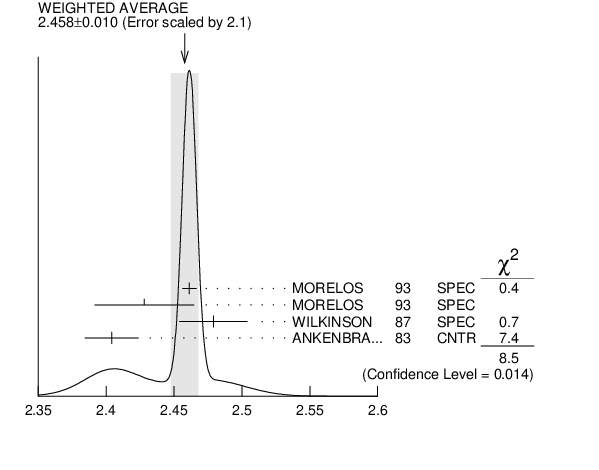# ${{\boldsymbol \Sigma}^{+}}$ MAGNETIC MOMENT INSPIRE search

See the Note on Baryon Magnetic Moments'' in the ${{\mathit \Lambda}}$ Listings. Measurements with an error ${}\geq{}$ $0.1$ ${\mathit \mu}_{{{\mathit N}}}$ have been omitted.
VALUE ($\mu _{\mathit N}$) EVTS DOCUMENT ID TECN  COMMENT
$\bf{ 2.458 \pm0.010}$ OUR AVERAGE  Error includes scale factor of 2.1.
$2.4613$ $\pm0.0034$ $\pm0.0040$ 250k
 1993
SPEC ${{\mathit p}}{}^{}\mathrm {Cu}$ 800 GeV
$2.428$ $\pm0.036$ $\pm0.007$ 12k 1
 1993
SPEC ${{\mathit p}}{}^{}\mathrm {Cu}$ 800 GeV
$2.479$ $\pm0.012$ $\pm0.022$ 137k
 1987
SPEC ${{\mathit p}}{}^{}\mathrm {Be}$ 400 GeV
$2.4040$ $\pm0.0198$ 44k 2
 1983
CNTR ${{\mathit p}}{}^{}\mathrm {Cu}$ 400 GeV
1  We assume $\mathit CPT$ invariance: this is (minus) the ${{\overline{\mathit \Sigma}}^{-}}$ magnetic moment as measured by MORELOS 1993 . See below for the moment difference testing $\mathit CPT$.
2  ANKENBRANDT 1983 gives the value $2.38$ $\pm0.02{\mathit \mu}_{{{\mathit N}}}$. MORELOS 1993 uses the same hyperon magnet and channel and claims to determine the field integral better, leading to the revised value given here.${{\mathit \Sigma}^{+}}$ magnetic moment ($\mu _{\mathit N}$)
References:
 MORELOS 1993
PRL 71 3417 Measurement of the Magnetic Moments of the ${{\mathit \Sigma}^{+}}$ and ${{\overline{\mathit \Sigma}}^{-}}$ Hyperons
 WILKINSON 1987
PRL 58 855 Polarization and Magnetic Moment of the ${{\mathit \Sigma}^{+}}$ Hyperon
 ANKENBRANDT 1983
PRL 51 863 Precise Measurement of the ${{\mathit \Sigma}^{+}}$ Magnetic Moment